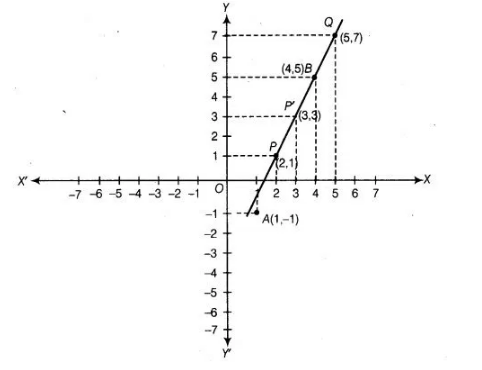# Plot the points A (1, – 1) and B (4, 5).`
Question:

Plot the points A (1, – 1) and B (4, 5).

(i) Draw the line segment joining these points. Write the coordinates of a point on this line segment between the points A and B.

(ii) Extend this line segment and write the coordinates of a point on this line which lies outside the line segment AB.

Solution:

In point A(1, -1), x-coordinate is positive and y-coordinate is negative, so it lies in IV quadrant. In point B(4, 5), both coordinates are positive, so it lies

in I quadrant. On plotting these point, we get the following graph.(i) On joining the points A and B, we get the line segment AB. Now, to find the coordinates of a point on this line segment between A and B draw a

perpendicular to X-axis from x = 2 and 3.

[since, x = 2 and 3 lies between A and B] say it intersect line segment AB at P and p’. Now, draw a perpendicular to Y-axis from P and p’, they

intersect Y-axis at y = 1 and 3, respectively. Thus, we get points (2,1) and (3, 3) which lie between line segment AB.

(ii) Extent the line segment AB. Now, draw a perpendicular to X-axis from x = 5, say it intersects extended line segment at Q. Again, draw a

perpendicular to Y-axis from Q and it intersects Y-axis at y = 7. Thus, we get the point Q(5,7) which lies outside the line segment AB.